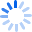# Machine Learning Vs. StatisticsThis article was written by Aatash Shah . Many people have this doubt, what’s the difference between statistics and machine learning? Is there something like machine learning vs. statistics? From a traditional data analytics standpoint, the answer to the above question is simple. - Machine Learning is an algorithm that can learn from data without relying on rules-based programming. - Statistical modeling is a formalization of relationships between variables in the data in the form of mathematical equations. Machine learning is all about predictions, supervised learning, unsupervised learning, etc. Statistics is about sample, population, hypothesis, etc. Two different critters, right? Well, let’s see if they are actually that different! Full story

18 AugustView all